Successfully reported this slideshow.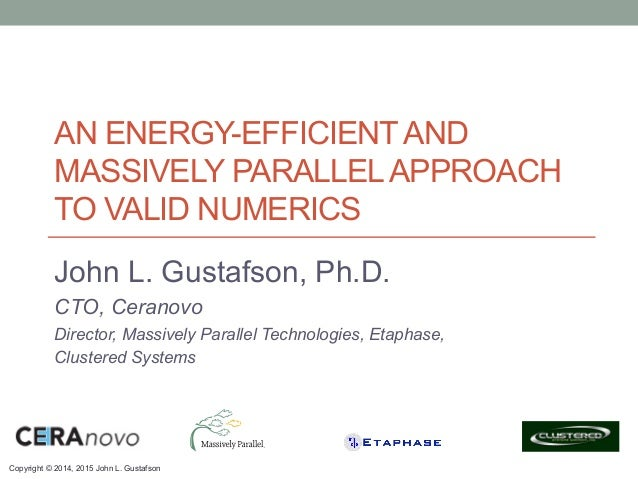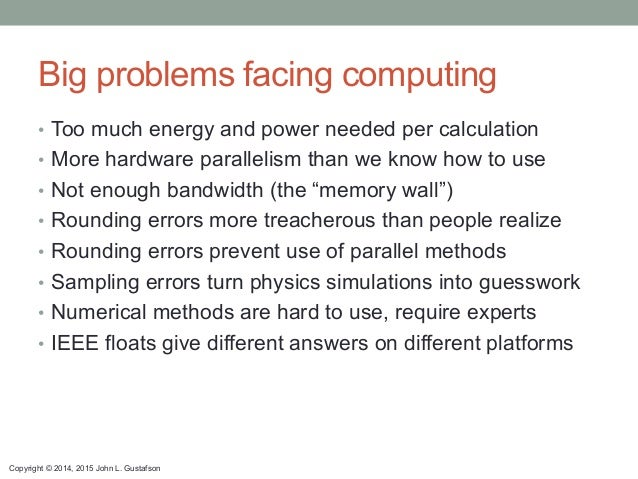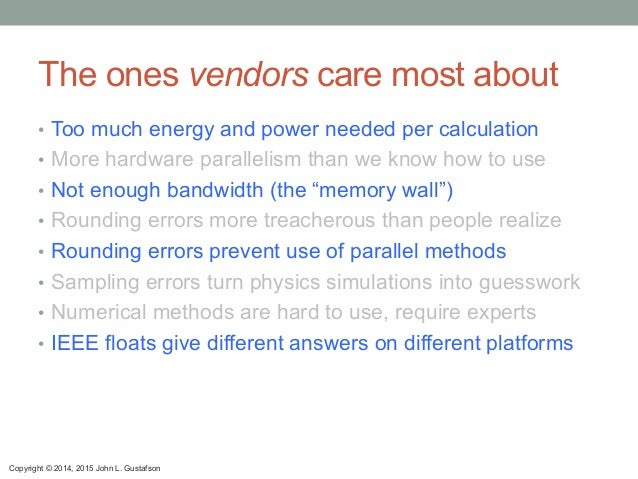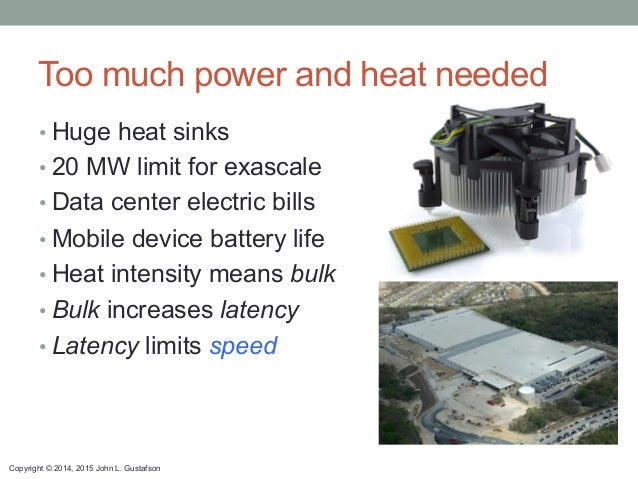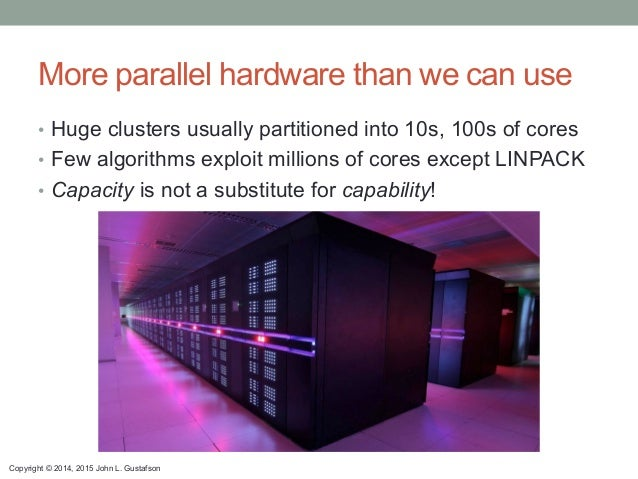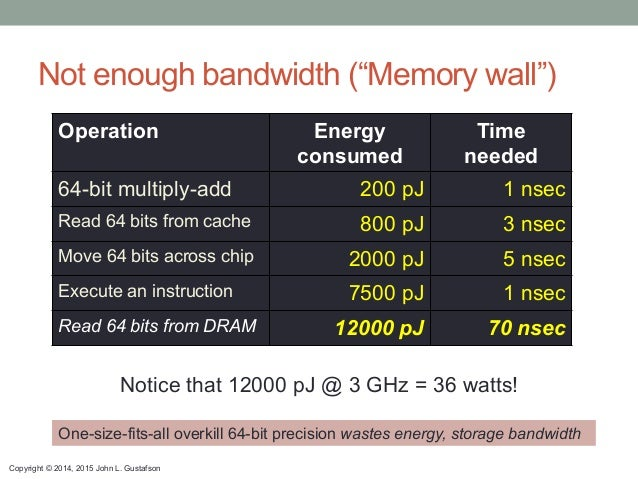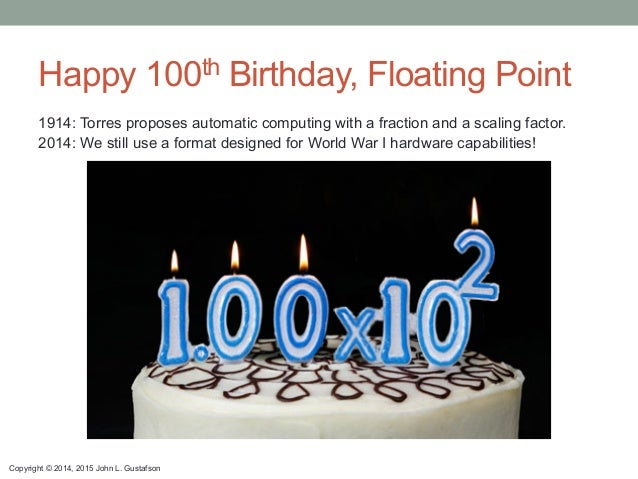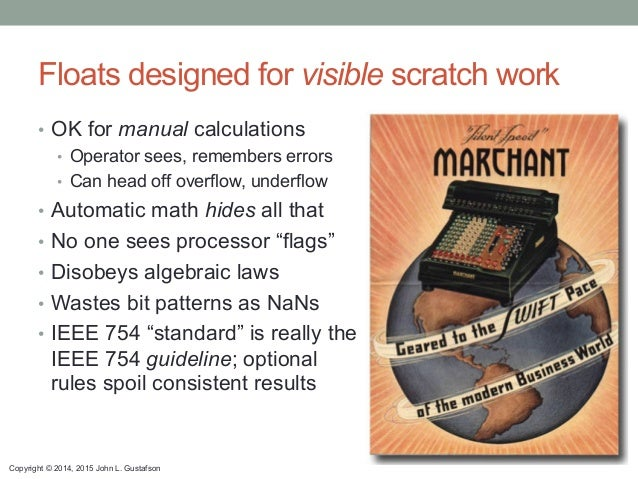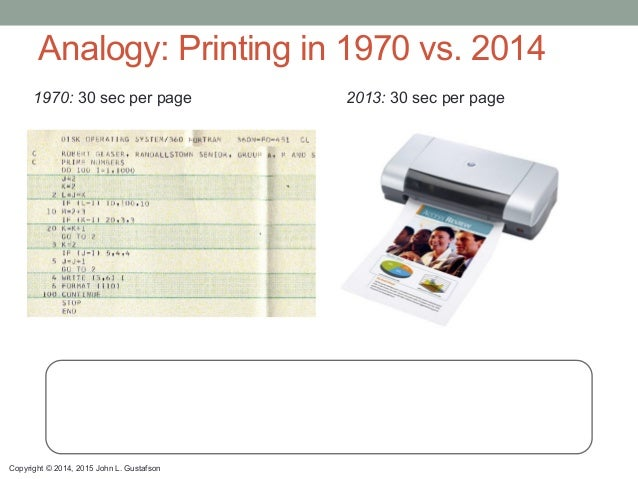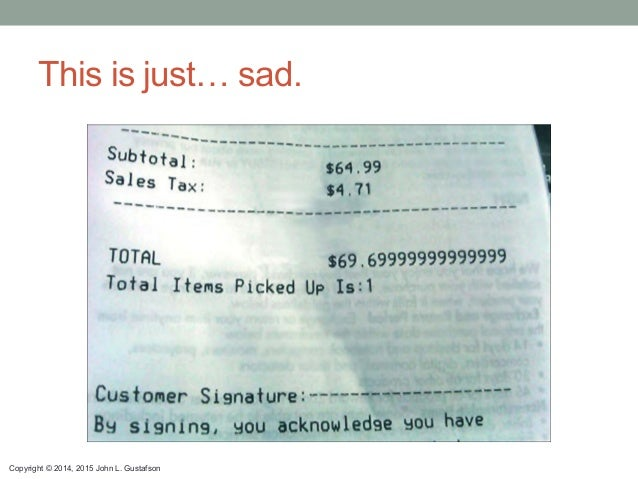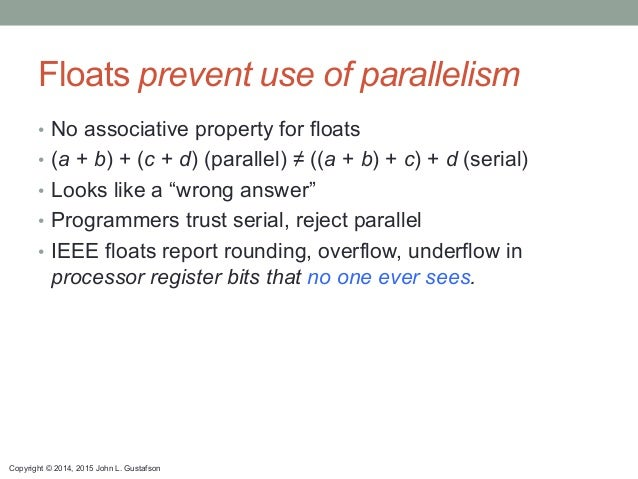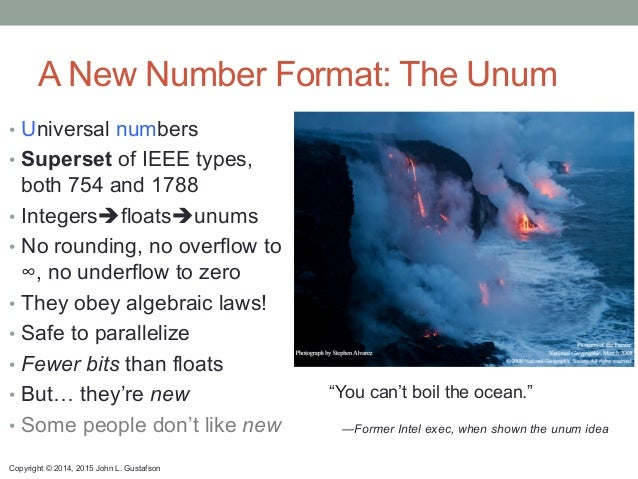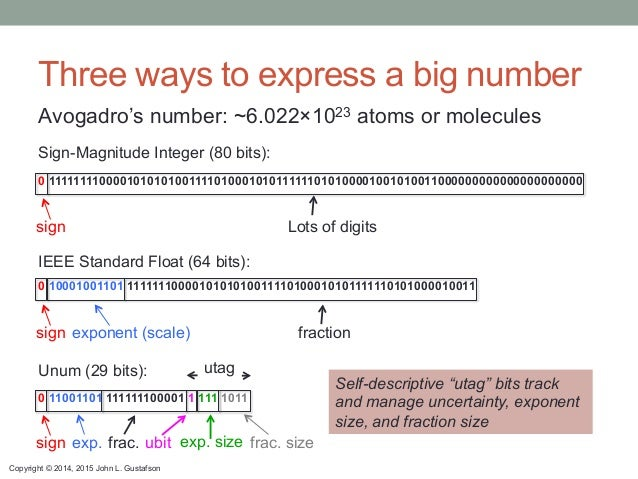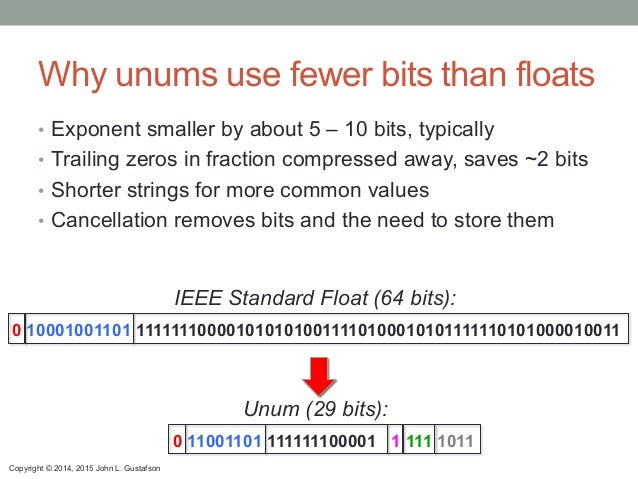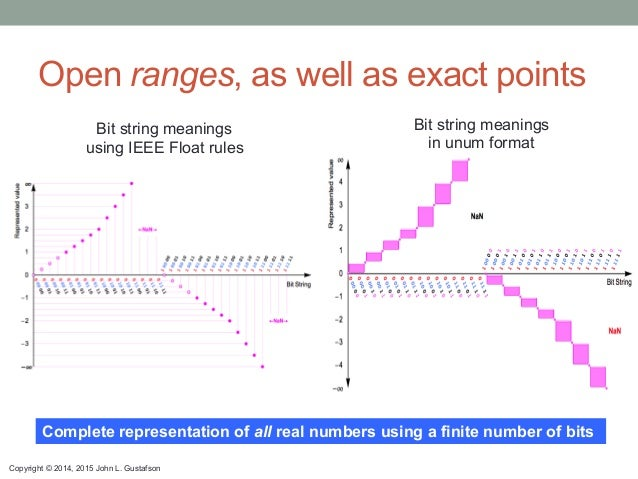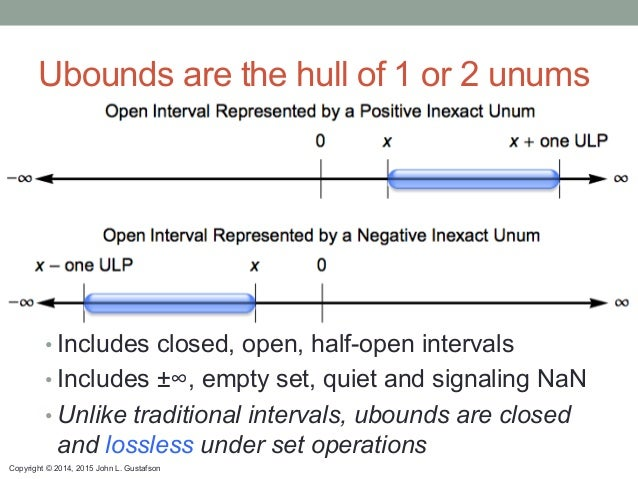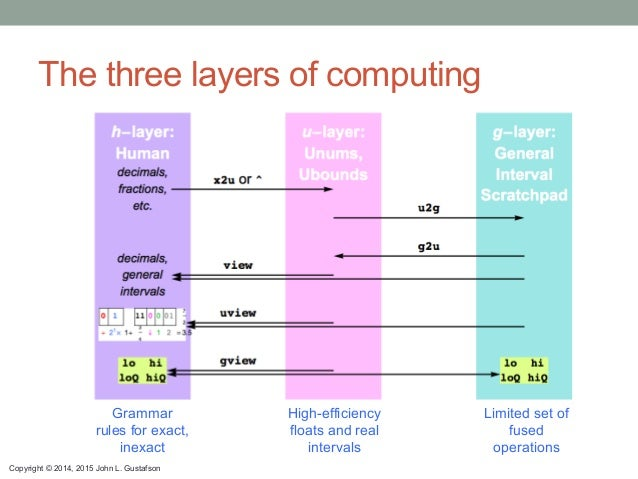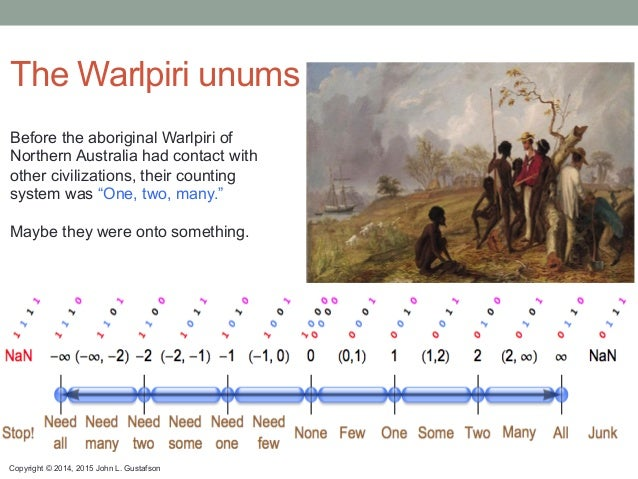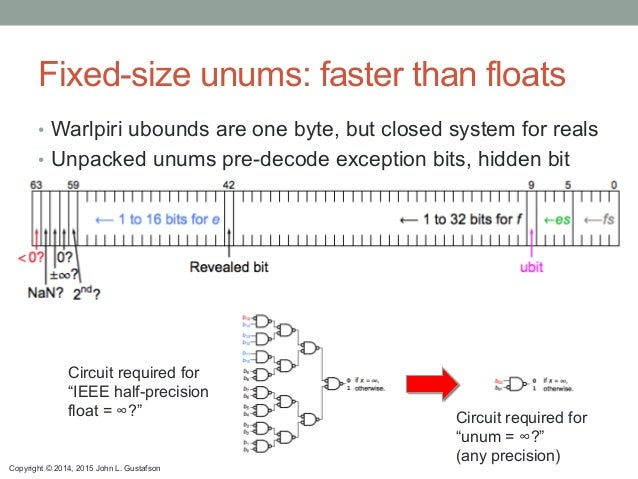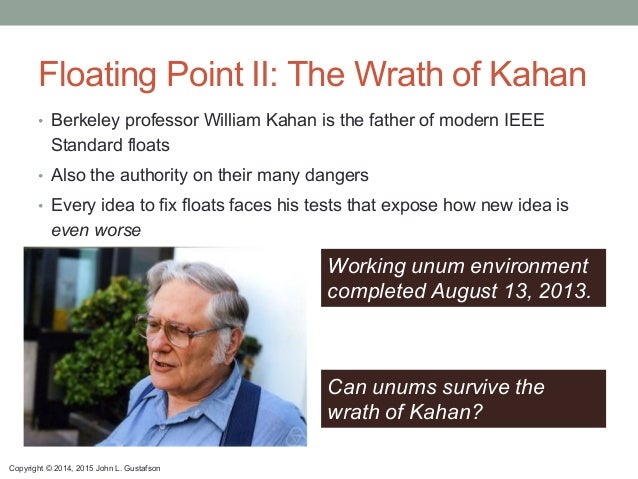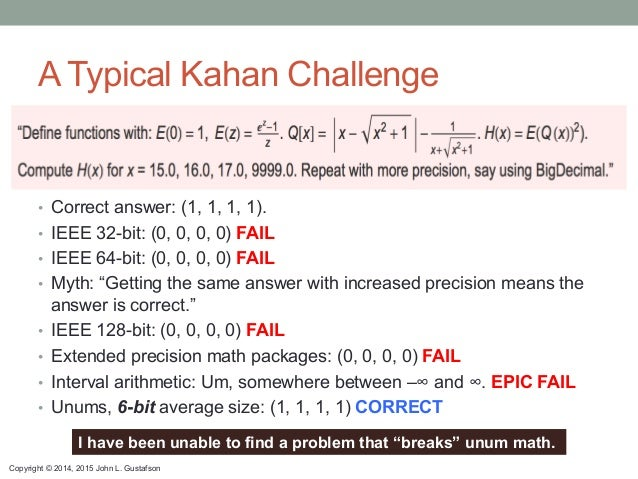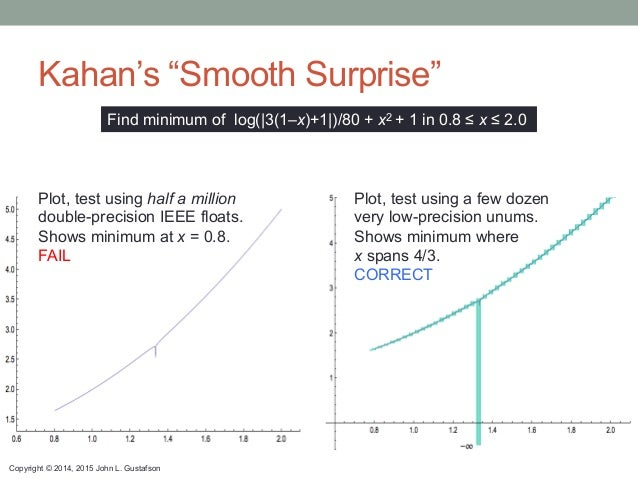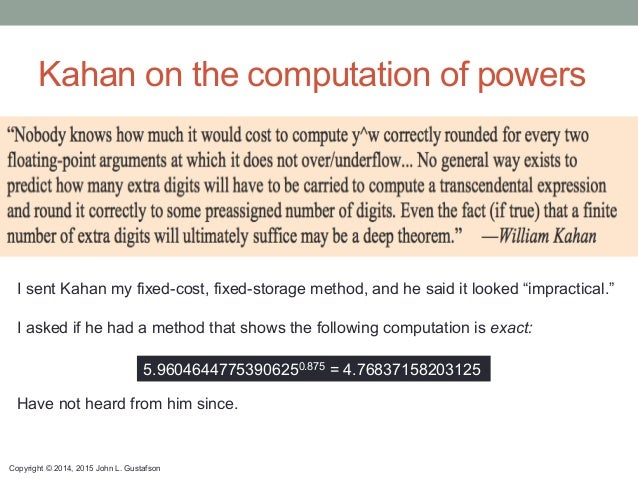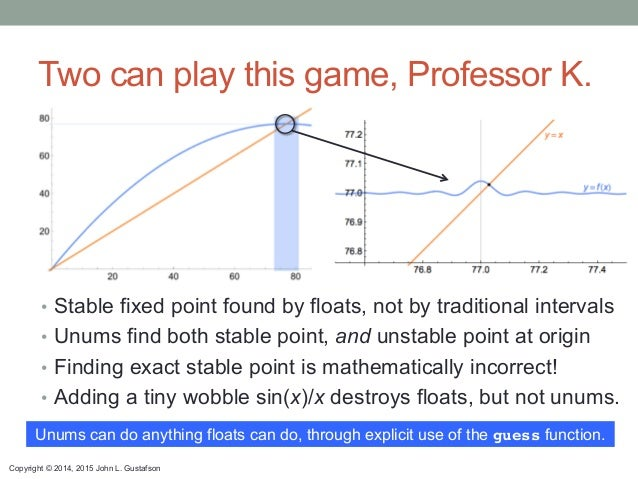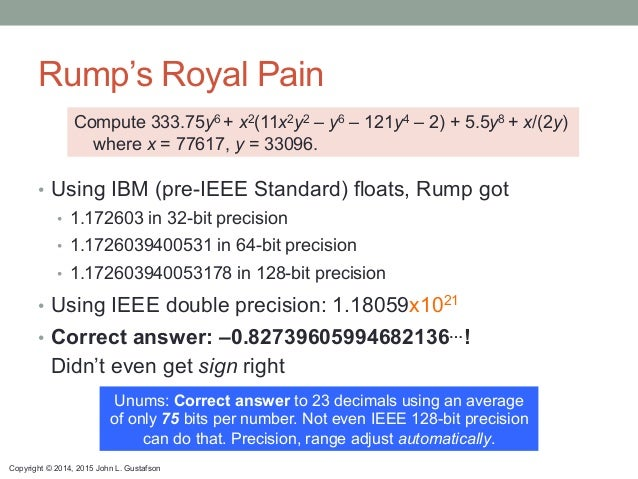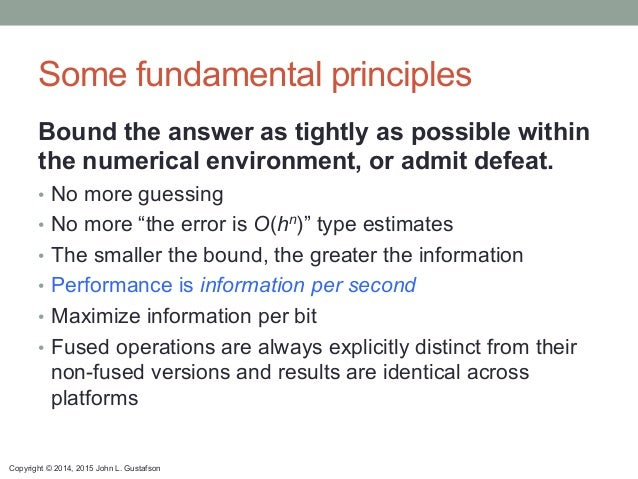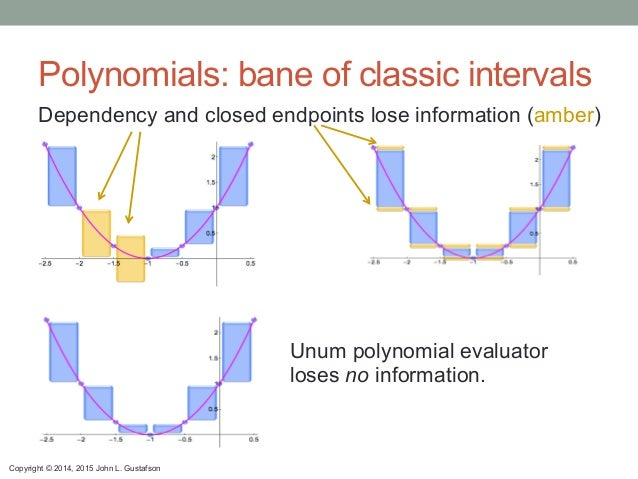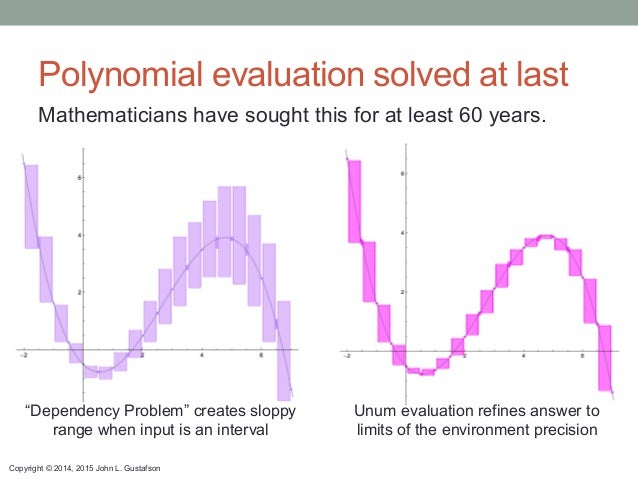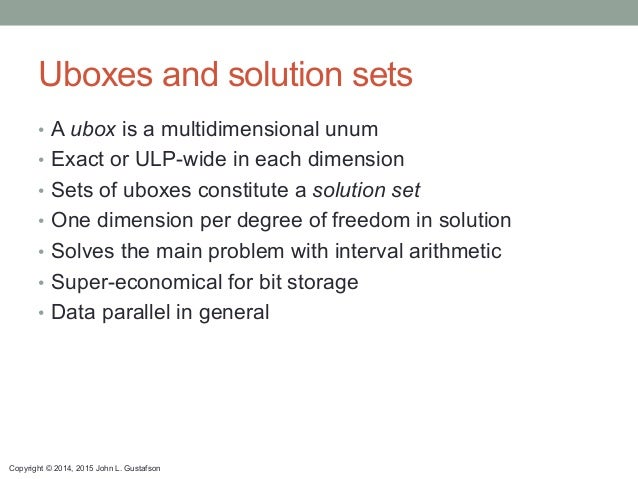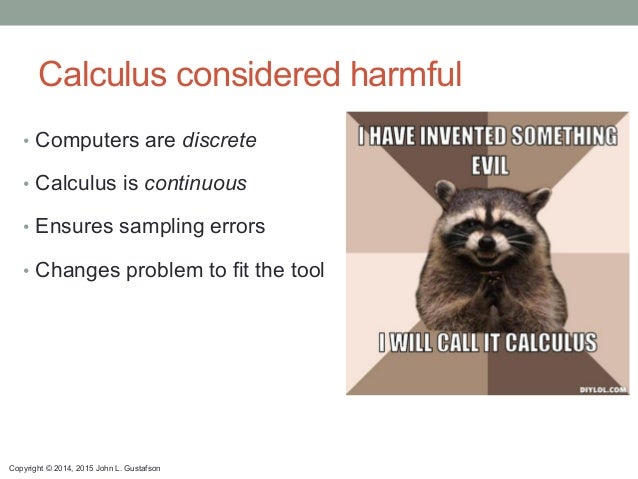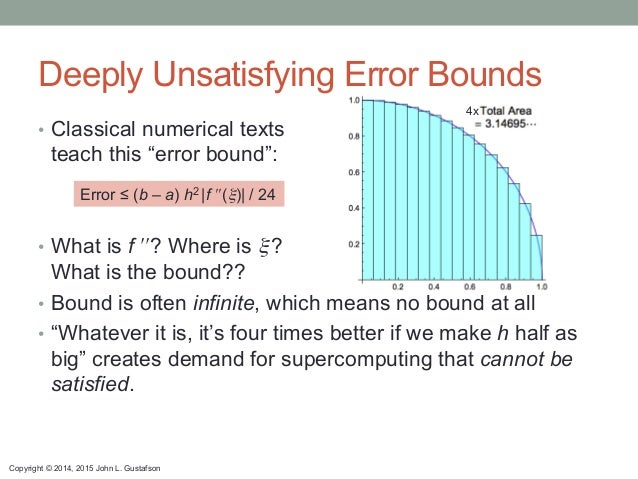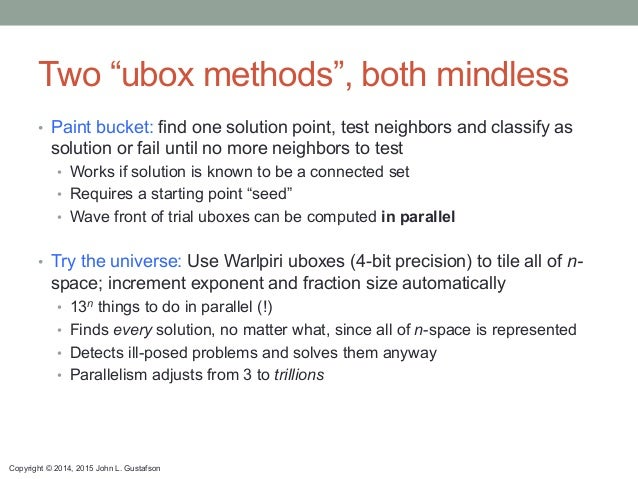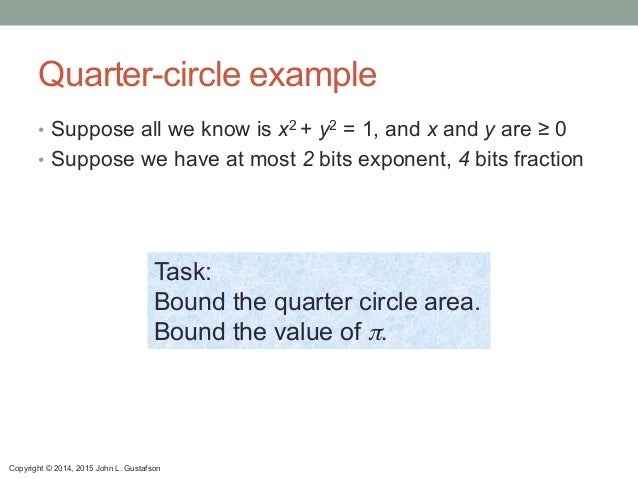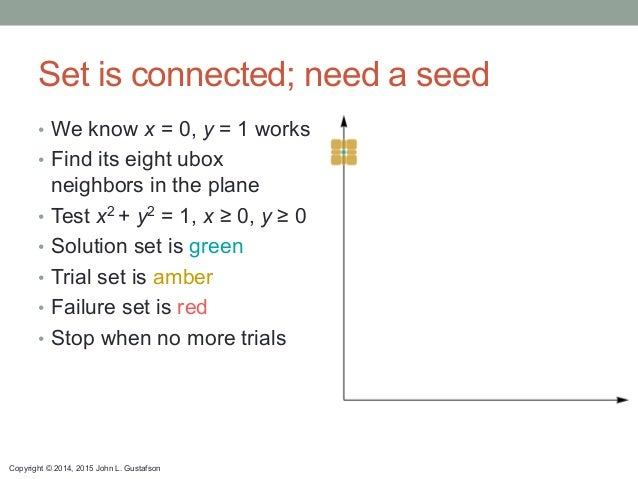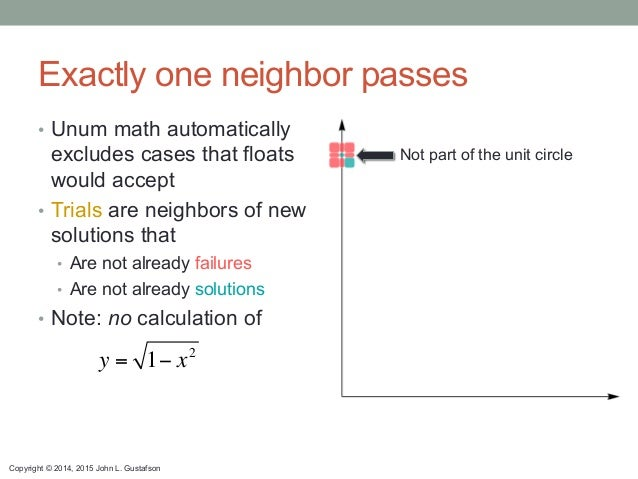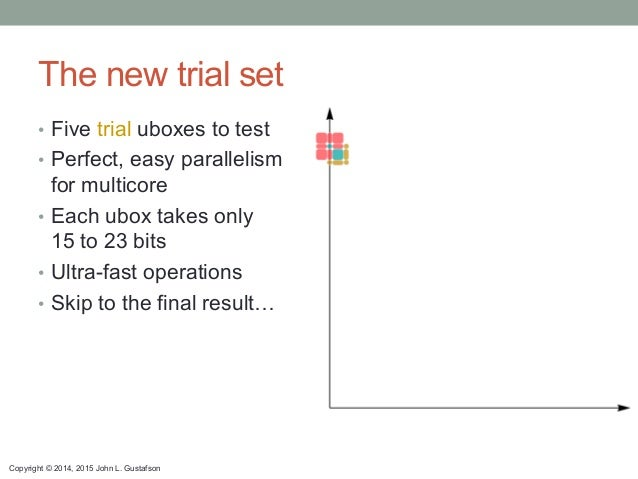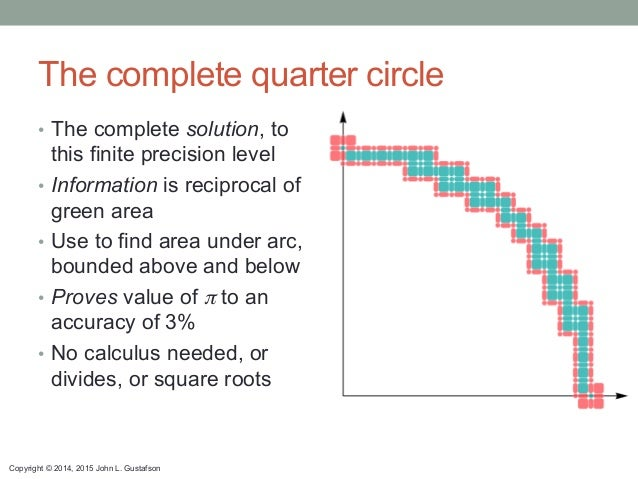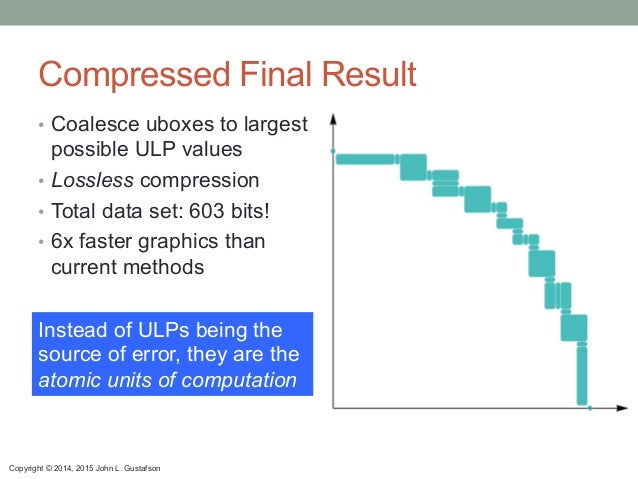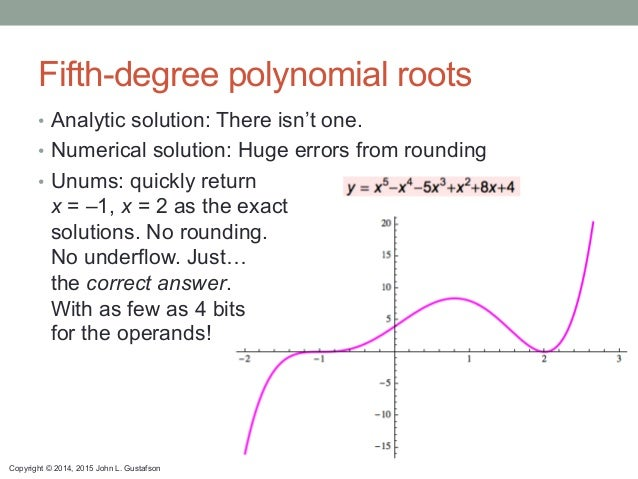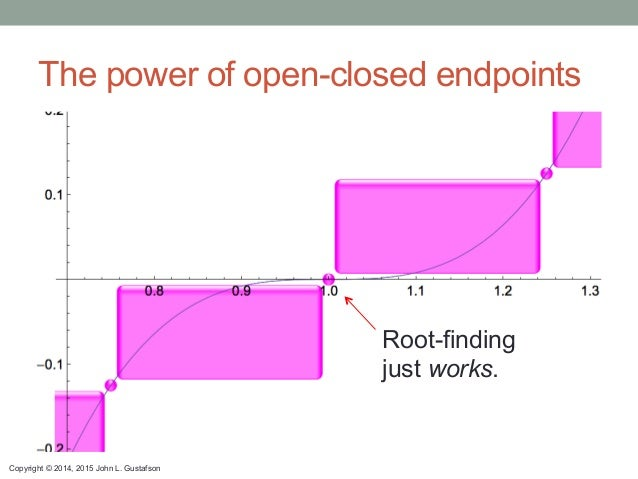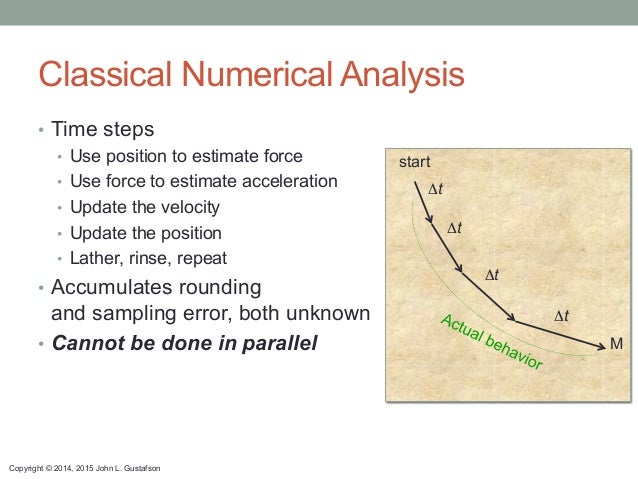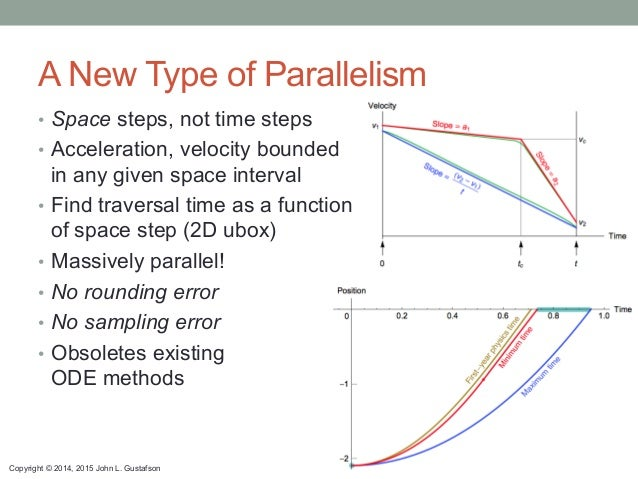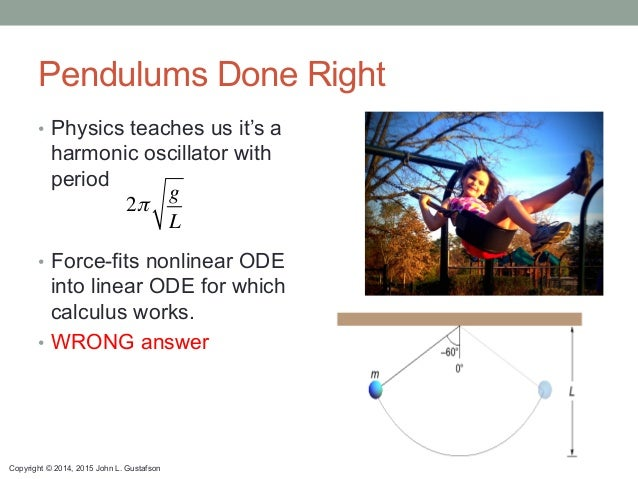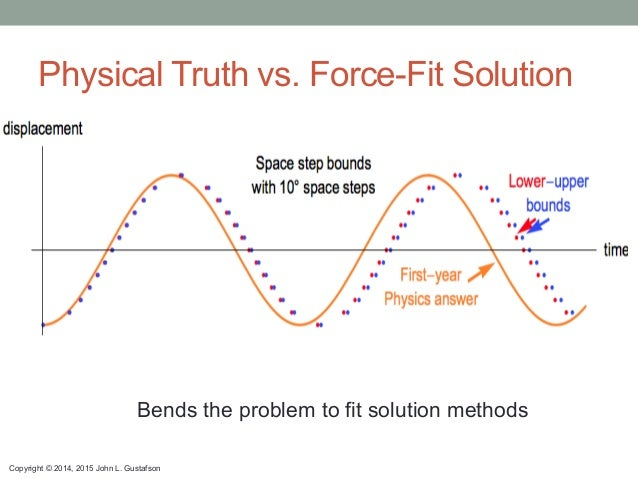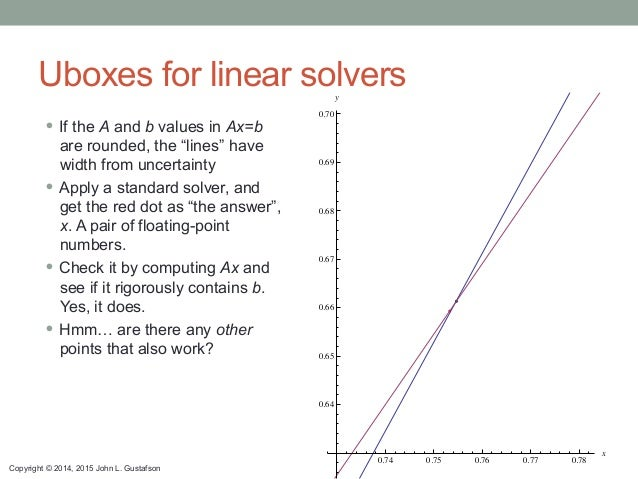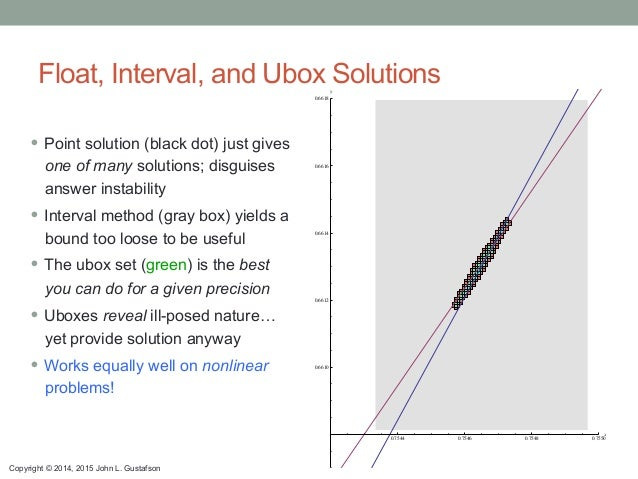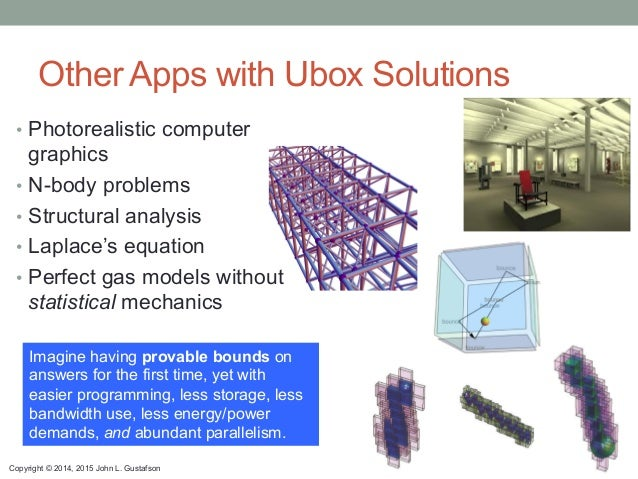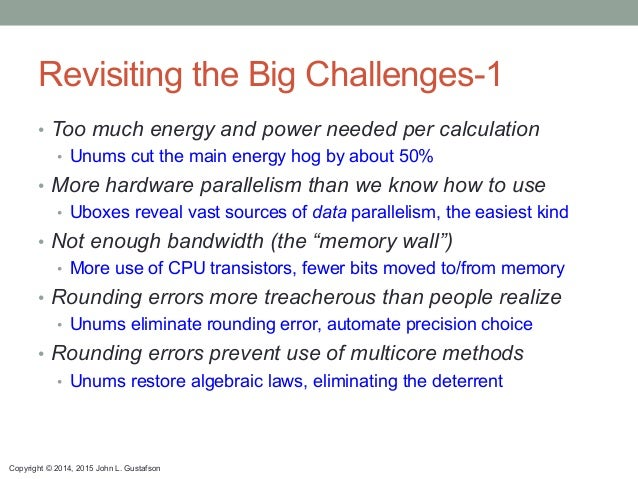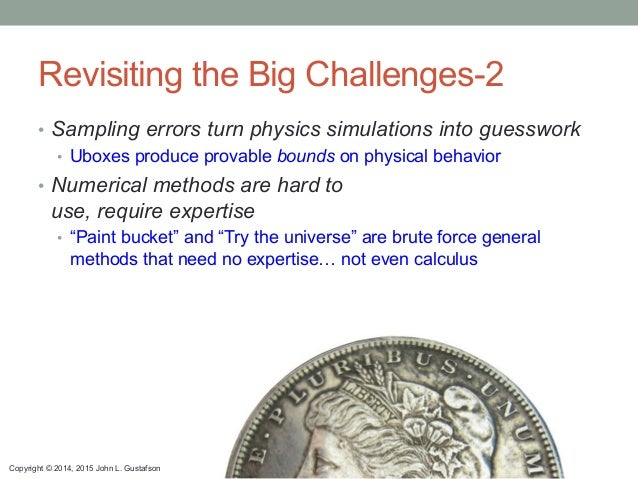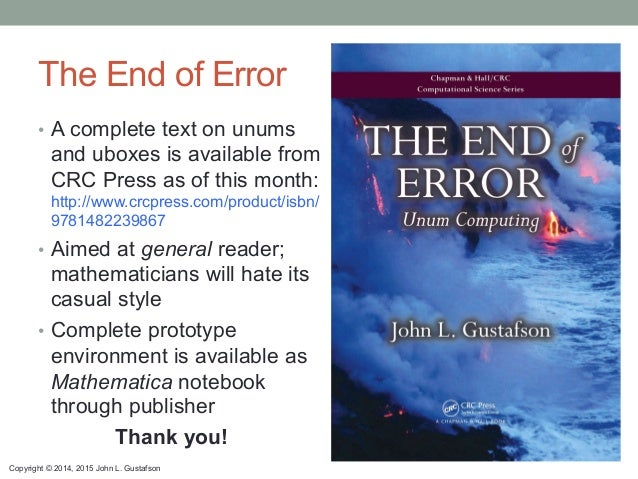Upcoming SlideShare
×

# Unum Computing: An Energy Efficient and Massively Parallel Approach to Valid Numerics

In this deck, John Gustafson presents: An Energy Efficient and Massively Parallel Approach to Valid Numerics.

"Written by one of the foremost experts in high-performance computing and the inventor of Gustafson’s Law, The End of Error: Unum Computing explains a new approach to computer arithmetic: the universal number (unum). The unum encompasses all IEEE floating-point formats as well as fixed-point and exact integer arithmetic. This new number type obtains more accurate answers than floating-point arithmetic yet uses fewer bits in many cases, saving memory, bandwidth, energy, and power."

Watch the video presentation: http://wp.me/p3RLHQ-dTk

• Full Name
Comment goes here.

Are you sure you want to Yes No• Be the first to comment

### Unum Computing: An Energy Efficient and Massively Parallel Approach to Valid Numerics

1. 1. Copyright © 2014, 2015 John L. Gustafson AN ENERGY-EFFICIENTAND MASSIVELY PARALLELAPPROACH TO VALID NUMERICS John L. Gustafson, Ph.D. CTO, Ceranovo Director, Massively Parallel Technologies, Etaphase, Clustered Systems
2. 2. Copyright © 2014, 2015 John L. Gustafson Big problems facing computing •  Too much energy and power needed per calculation •  More hardware parallelism than we know how to use •  Not enough bandwidth (the “memory wall”) •  Rounding errors more treacherous than people realize •  Rounding errors prevent use of parallel methods •  Sampling errors turn physics simulations into guesswork •  Numerical methods are hard to use, require experts •  IEEE floats give different answers on different platforms
3. 3. Copyright © 2014, 2015 John L. Gustafson The ones vendors care most about •  Too much energy and power needed per calculation •  More hardware parallelism than we know how to use •  Not enough bandwidth (the “memory wall”) •  Rounding errors more treacherous than people realize •  Rounding errors prevent use of parallel methods •  Sampling errors turn physics simulations into guesswork •  Numerical methods are hard to use, require experts •  IEEE floats give different answers on different platforms
4. 4. Copyright © 2014, 2015 John L. Gustafson Too much power and heat needed • Huge heat sinks • 20 MW limit for exascale • Data center electric bills • Mobile device battery life • Heat intensity means bulk • Bulk increases latency • Latency limits speed
5. 5. Copyright © 2014, 2015 John L. Gustafson More parallel hardware than we can use •  Huge clusters usually partitioned into 10s, 100s of cores •  Few algorithms exploit millions of cores except LINPACK •  Capacity is not a substitute for capability!
6. 6. Copyright © 2014, 2015 John L. Gustafson Not enough bandwidth (“Memory wall”) Operation Energy consumed Time needed 64-bit multiply-add 200 pJ 1 nsec Read 64 bits from cache 800 pJ 3 nsec Move 64 bits across chip 2000 pJ 5 nsec Execute an instruction 7500 pJ 1 nsec Read 64 bits from DRAM 12000 pJ 70 nsec Notice that 12000 pJ @ 3 GHz = 36 watts! One-size-fits-all overkill 64-bit precision wastes energy, storage bandwidth
7. 7. Copyright © 2014, 2015 John L. Gustafson Happy 100th Birthday, Floating Point 1914: Torres proposes automatic computing with a fraction and a scaling factor. 2014: We still use a format designed for World War I hardware capabilities!
8. 8. Copyright © 2014, 2015 John L. Gustafson Floats designed for visible scratch work •  OK for manual calculations •  Operator sees, remembers errors •  Can head off overflow, underflow •  Automatic math hides all that •  No one sees processor “flags” •  Disobeys algebraic laws •  Wastes bit patterns as NaNs •  IEEE 754 “standard” is really the IEEE 754 guideline; optional rules spoil consistent results
9. 9. Copyright © 2014, 2015 John L. Gustafson Analogy: Printing in 1970 vs. 2014 1970: 30 sec per page 2013: 30 sec per page Faster technology is for better prints, not thousands of low-quality prints per second. Why not do the same thing with computer arithmetic?
11. 11. Copyright © 2014, 2015 John L. Gustafson Floats prevent use of parallelism •  No associative property for floats •  (a + b) + (c + d) (parallel) ≠ ((a + b) + c) + d (serial) •  Looks like a “wrong answer” •  Programmers trust serial, reject parallel •  IEEE floats report rounding, overflow, underflow in processor register bits that no one ever sees.
12. 12. Copyright © 2014, 2015 John L. Gustafson A New Number Format: The Unum •  Universal numbers •  Superset of IEEE types, both 754 and 1788 •  Integersèfloatsèunums •  No rounding, no overflow to ∞, no underflow to zero •  They obey algebraic laws! •  Safe to parallelize •  Fewer bits than floats •  But… they’re new •  Some people don’t like new “You can’t boil the ocean.” —Former Intel exec, when shown the unum idea
13. 13. Copyright © 2014, 2015 John L. Gustafson Three ways to express a big number Avogadro’s number: ~6.022×1023 atoms or molecules Unum (29 bits): 0 11001101 111111100001 1 111 1011 sign exp. frac. ubit exp. size frac. size Self-descriptive “utag” bits track and manage uncertainty, exponent size, and fraction size utag IEEE Standard Float (64 bits): 0 10001001101 1111111000010101010011110100010101111110101000010011 sign exponent (scale) fraction Sign-Magnitude Integer (80 bits): 0 1111111100001010101001111010001010111111010100001001010011000000000000000000000 sign Lots of digits
14. 14. Copyright © 2014, 2015 John L. Gustafson Why unums use fewer bits than floats •  Exponent smaller by about 5 – 10 bits, typically •  Trailing zeros in fraction compressed away, saves ~2 bits •  Shorter strings for more common values •  Cancellation removes bits and the need to store them Unum (29 bits): IEEE Standard Float (64 bits): 0 10001001101 1111111000010101010011110100010101111110101000010011 0 11001101 111111100001 1 111 1011
15. 15. Copyright © 2014, 2015 John L. Gustafson Open ranges, as well as exact points Bit string meanings using IEEE Float rules Bit string meanings in unum format Complete representation of all real numbers using a finite number of bits
16. 16. Copyright © 2014, 2015 John L. Gustafson Ubounds are the hull of 1 or 2 unums • Includes closed, open, half-open intervals • Includes ±∞, empty set, quiet and signaling NaN • Unlike traditional intervals, ubounds are closed and lossless under set operations
17. 17. Copyright © 2014, 2015 John L. Gustafson The three layers of computing Grammar rules for exact, inexact High-efficiency floats and real intervals Limited set of fused operations
18. 18. Copyright © 2014, 2015 John L. Gustafson The Warlpiri unums Before the aboriginal Warlpiri of Northern Australia had contact with other civilizations, their counting system was “One, two, many.” Maybe they were onto something.
19. 19. Copyright © 2014, 2015 John L. Gustafson Fixed-size unums: faster than floats •  Warlpiri ubounds are one byte, but closed system for reals •  Unpacked unums pre-decode exception bits, hidden bit Circuit required for “IEEE half-precision float = ∞?” Circuit required for “unum = ∞?” (any precision)
20. 20. Copyright © 2014, 2015 John L. Gustafson Floating Point II: The Wrath of Kahan •  Berkeley professor William Kahan is the father of modern IEEE Standard floats •  Also the authority on their many dangers •  Every idea to fix floats faces his tests that expose how new idea is even worse Working unum environment completed August 13, 2013. Can unums survive the wrath of Kahan?
21. 21. Copyright © 2014, 2015 John L. Gustafson A Typical Kahan Challenge •  Correct answer: (1, 1, 1, 1). •  IEEE 32-bit: (0, 0, 0, 0) FAIL •  IEEE 64-bit: (0, 0, 0, 0) FAIL •  Myth: “Getting the same answer with increased precision means the answer is correct.” •  IEEE 128-bit: (0, 0, 0, 0) FAIL •  Extended precision math packages: (0, 0, 0, 0) FAIL •  Interval arithmetic: Um, somewhere between –∞ and ∞. EPIC FAIL •  Unums, 6-bit average size: (1, 1, 1, 1) CORRECT I have been unable to find a problem that “breaks” unum math.
22. 22. Copyright © 2014, 2015 John L. Gustafson Kahan’s “Smooth Surprise” Find minimum of log(|3(1–x)+1|)/80 + x2 + 1 in 0.8 ≤ x ≤ 2.0 Plot, test using half a million double-precision IEEE floats. Shows minimum at x = 0.8. FAIL Plot, test using a few dozen very low-precision unums. Shows minimum where x spans 4/3. CORRECT
23. 23. Copyright © 2014, 2015 John L. Gustafson Kahan on the computation of powers 5.96046447753906250.875 = 4.76837158203125 I sent Kahan my fixed-cost, fixed-storage method, and he said it looked “impractical.” I asked if he had a method that shows the following computation is exact: Have not heard from him since.
24. 24. Copyright © 2014, 2015 John L. Gustafson Two can play this game, Professor K. Unums can do anything floats can do, through explicit use of the guess function. •  Stable fixed point found by floats, not by traditional intervals •  Unums find both stable point, and unstable point at origin •  Finding exact stable point is mathematically incorrect! •  Adding a tiny wobble sin(x)/x destroys floats, but not unums.
25. 25. Copyright © 2014, 2015 John L. Gustafson Rump’s Royal Pain •  Using IBM (pre-IEEE Standard) floats, Rump got •  1.172603 in 32-bit precision •  1.1726039400531 in 64-bit precision •  1.172603940053178 in 128-bit precision •  Using IEEE double precision: 1.18059x1021 •  Correct answer: –0.82739605994682136…! Didn’t even get sign right Compute 333.75y6 + x2(11x2y2 – y6 – 121y4 – 2) + 5.5y8 + x/(2y) where x = 77617, y = 33096. Unums: Correct answer to 23 decimals using an average of only 75 bits per number. Not even IEEE 128-bit precision can do that. Precision, range adjust automatically.
26. 26. Copyright © 2014, 2015 John L. Gustafson Some fundamental principles Bound the answer as tightly as possible within the numerical environment, or admit defeat. •  No more guessing •  No more “the error is O(hn)” type estimates •  The smaller the bound, the greater the information •  Performance is information per second •  Maximize information per bit •  Fused operations are always explicitly distinct from their non-fused versions and results are identical across platforms
27. 27. Copyright © 2014, 2015 John L. Gustafson Polynomials: bane of classic intervals Dependency and closed endpoints lose information (amber) Unum polynomial evaluator loses no information.
28. 28. Copyright © 2014, 2015 John L. Gustafson Polynomial evaluation solved at last Mathematicians have sought this for at least 60 years. “Dependency Problem” creates sloppy range when input is an interval Unum evaluation refines answer to limits of the environment precision
29. 29. Copyright © 2014, 2015 John L. Gustafson Uboxes and solution sets •  A ubox is a multidimensional unum •  Exact or ULP-wide in each dimension •  Sets of uboxes constitute a solution set •  One dimension per degree of freedom in solution •  Solves the main problem with interval arithmetic •  Super-economical for bit storage •  Data parallel in general
30. 30. Copyright © 2014, 2015 John L. Gustafson Calculus considered harmful •  Computers are discrete •  Calculus is continuous •  Ensures sampling errors •  Changes problem to fit the tool
31. 31. Copyright © 2014, 2015 John L. Gustafson Deeply Unsatisfying Error Bounds Error ≤ (b – a) h2 |f ʹ′ʹ′(ξ)| / 24 •  Classical numerical texts teach this “error bound”: •  What is f ʹ′ʹ′? Where is ξ? What is the bound?? •  Bound is often infinite, which means no bound at all •  “Whatever it is, it’s four times better if we make h half as big” creates demand for supercomputing that cannot be satisfied. 4x
32. 32. Copyright © 2014, 2015 John L. Gustafson Two “ubox methods”, both mindless •  Paint bucket: find one solution point, test neighbors and classify as solution or fail until no more neighbors to test •  Works if solution is known to be a connected set •  Requires a starting point “seed” •  Wave front of trial uboxes can be computed in parallel •  Try the universe: Use Warlpiri uboxes (4-bit precision) to tile all of n- space; increment exponent and fraction size automatically •  13n things to do in parallel (!) •  Finds every solution, no matter what, since all of n-space is represented •  Detects ill-posed problems and solves them anyway •  Parallelism adjusts from 3 to trillions
33. 33. Copyright © 2014, 2015 John L. Gustafson Quarter-circle example •  Suppose all we know is x2 + y2 = 1, and x and y are ≥ 0 •  Suppose we have at most 2 bits exponent, 4 bits fraction Task: Bound the quarter circle area. Bound the value of π.
34. 34. Copyright © 2014, 2015 John L. Gustafson Set is connected; need a seed •  We know x = 0, y = 1 works •  Find its eight ubox neighbors in the plane •  Test x2 + y2 = 1, x ≥ 0, y ≥ 0 •  Solution set is green •  Trial set is amber •  Failure set is red •  Stop when no more trials
35. 35. Copyright © 2014, 2015 John L. Gustafson Exactly one neighbor passes •  Unum math automatically excludes cases that floats would accept •  Trials are neighbors of new solutions that •  Are not already failures •  Are not already solutions •  Note: no calculation of Not part of the unit circle y = 1− x2
36. 36. Copyright © 2014, 2015 John L. Gustafson The new trial set •  Five trial uboxes to test •  Perfect, easy parallelism for multicore •  Each ubox takes only 15 to 23 bits •  Ultra-fast operations •  Skip to the final result…
37. 37. Copyright © 2014, 2015 John L. Gustafson The complete quarter circle •  The complete solution, to this finite precision level •  Information is reciprocal of green area •  Use to find area under arc, bounded above and below •  Proves value of π to an accuracy of 3% •  No calculus needed, or divides, or square roots
38. 38. Copyright © 2014, 2015 John L. Gustafson Compressed Final Result •  Coalesce uboxes to largest possible ULP values •  Lossless compression •  Total data set: 603 bits! •  6x faster graphics than current methods Instead of ULPs being the source of error, they are the atomic units of computation
39. 39. Copyright © 2014, 2015 John L. Gustafson Fifth-degree polynomial roots •  Analytic solution: There isn’t one. •  Numerical solution: Huge errors from rounding •  Unums: quickly return x = –1, x = 2 as the exact solutions. No rounding. No underflow. Just… the correct answer. With as few as 4 bits for the operands!
40. 40. Copyright © 2014, 2015 John L. Gustafson The power of open-closed endpoints Root-finding just works.
41. 41. Copyright © 2014, 2015 John L. Gustafson Classical Numerical Analysis •  Time steps •  Use position to estimate force •  Use force to estimate acceleration •  Update the velocity •  Update the position •  Lather, rinse, repeat •  Accumulates rounding and sampling error, both unknown •  Cannot be done in parallel start Δt M Δt Δt Δt
42. 42. Copyright © 2014, 2015 John L. Gustafson A New Type of Parallelism •  Space steps, not time steps •  Acceleration, velocity bounded in any given space interval •  Find traversal time as a function of space step (2D ubox) •  Massively parallel! •  No rounding error •  No sampling error •  Obsoletes existing ODE methods
43. 43. Copyright © 2014, 2015 John L. Gustafson Pendulums Done Right •  Physics teaches us it’s a harmonic oscillator with period •  Force-fits nonlinear ODE into linear ODE for which calculus works. •  WRONG answer 2π g L
44. 44. Copyright © 2014, 2015 John L. Gustafson Physical Truth vs. Force-Fit Solution Bends the problem to fit solution methods
45. 45. Copyright © 2014, 2015 John L. Gustafson Uboxes for linear solvers 0.74 0.75 0.76 0.77 0.78 x 0.64 0.65 0.66 0.67 0.68 0.69 0.70 y • If the A and b values in Ax=b are rounded, the “lines” have width from uncertainty • Apply a standard solver, and get the red dot as “the answer”, x. A pair of floating-point numbers. • Check it by computing Ax and see if it rigorously contains b. Yes, it does. • Hmm… are there any other points that also work?
46. 46. Copyright © 2014, 2015 John L. Gustafson Float, Interval, and Ubox Solutions 0.7544 0.7546 0.7548 0.7550 x 0.6610 0.6612 0.6614 0.6616 0.6618 y • Point solution (black dot) just gives one of many solutions; disguises answer instability • Interval method (gray box) yields a bound too loose to be useful • The ubox set (green) is the best you can do for a given precision • Uboxes reveal ill-posed nature… yet provide solution anyway • Works equally well on nonlinear problems!
47. 47. Copyright © 2014, 2015 John L. Gustafson Other Apps with Ubox Solutions Imagine having provable bounds on answers for the first time, yet with easier programming, less storage, less bandwidth use, less energy/power demands, and abundant parallelism. •  Photorealistic computer graphics •  N-body problems •  Structural analysis •  Laplace’s equation •  Perfect gas models without statistical mechanics
48. 48. Copyright © 2014, 2015 John L. Gustafson Revisiting the Big Challenges-1 •  Too much energy and power needed per calculation •  Unums cut the main energy hog by about 50% •  More hardware parallelism than we know how to use •  Uboxes reveal vast sources of data parallelism, the easiest kind •  Not enough bandwidth (the “memory wall”) •  More use of CPU transistors, fewer bits moved to/from memory •  Rounding errors more treacherous than people realize •  Unums eliminate rounding error, automate precision choice •  Rounding errors prevent use of multicore methods •  Unums restore algebraic laws, eliminating the deterrent
49. 49. Copyright © 2014, 2015 John L. Gustafson Revisiting the Big Challenges-2 •  Sampling errors turn physics simulations into guesswork •  Uboxes produce provable bounds on physical behavior •  Numerical methods are hard to use, require expertise •  “Paint bucket” and “Try the universe” are brute force general methods that need no expertise… not even calculus
50. 50. Copyright © 2014, 2015 John L. Gustafson The End of Error •  A complete text on unums and uboxes is available from CRC Press as of this month: http://www.crcpress.com/product/isbn/ 9781482239867 •  Aimed at general reader; mathematicians will hate its casual style •  Complete prototype environment is available as Mathematica notebook through publisher Thank you!